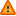FAQ/percentile - CBU statistics WikiYou should trust both wikis because the password could be read by the particular administrators.

Clear message
location: FAQ / percentile

## Percentiles of exponential data and use in outlier detection

If the data follows an exponential distribution (test this by using Kolmogorov-Smirnov test in SPSS and choosing the exponential option). Then

- (Mean score) (LN(1- PERC/100 )) gives the threshold of the PERC% percentile.

This follows from

P(X < x) = 1 – EXP(-R*x) where rate, R, is estimated from the sample data by 1/mean.

These percentiles may be used to classify a data point as extreme since outliers are defined as either

> 75th percentile + 1.5 * (75th perc - 25th perc)

or

< 25th percentile - 1.5 * (75th perc - 25th perc)